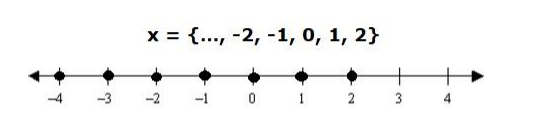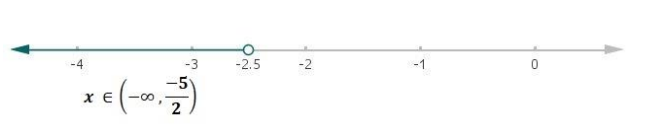# Solve each of the following in equations and represent the solution set on

Question:

Solve each of the following in equations and represent the solution set on the number line.

$-2 x>5$, where (i) $x \in Z$, (ii) $x \in R$.

Solution:

(i) $-2 x>5, x \in Z$

Multiply both the sides by -1 in above equation,

$-2 x(-1)>5(-1)$

$2 x<-5$

Dividing both the sides by 2 in above equation,

$\frac{2 x}{2}<\frac{-5}{2}$

$x<\frac{-5}{2}$

$x<2.5$

Since, x is an integer

Therefore, possible values of x can be

$x=\{\ldots,-2,-1,0,1,2\}$(ii) $-2 x>5, x \in R$

Multiply both the sides by -1 in above equation,

$-2 x(-1)>5(-1)$

$2 x<-5$

Dividing both the sides by 2 in above equation,

$\frac{2 x}{2}<\frac{-5}{2}$

$x<\frac{-5}{2}$

Therefore,

$x \in\left(-\infty, \frac{-5}{2}\right)$